# FAQ: Why do the two terms for the rate of change of the congestion control window size in equation (3.16) have different units?

Jump to: navigation, search

(Contributed by Richard Murray, 15 November 2008)

The dynamics of the window size for the TCP/Reno congestion control protocol are given in equation (3.16):where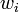is the window size for source,is the end-to-end probability that a packet is dropped,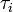is the end-to-end transmission time for a packet to reach its destination and the acknowledgement to be sent back and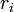is the resulting rate at which packets are cleared from the list of packets that have been received.

The first term in this expression has units ofand the second term has units of. The left-hand side of the equation is the rate of change of the window size, which has units pkts/sec. This lack of consistence in units results from some explicit gain factors in each of the terms. Suppose we rewrite the window dynamics as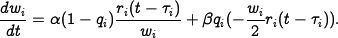• For the rate at which we increase the window size, we have that "the window size is increased by 1 every time a packet is acknowledged". The percentage of packets that are received per unit time is given by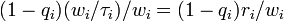. For each fractional packet that is acknowledged (per unit time), we increase the buffer size by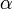packets. The default value ofis 1 [pkt].
• Similarly, we have that "the window size is cut in half when packets are lost". The number of packets that are lost per unit time is given by the rate at which ack packets are received (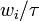) times the probability of loss (. This time we want to decrease the window size not by an absolute number, but by a fraction of its current size. Hence we decrease the buffer size byfor each packet lost per unit time, giving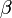the units of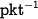. The default value ofis 1/2 [].